Egwald Mathematics: Nonlinear Dynamics:

Limit Cycles and Two Dimensional Flows

by

Elmer G. Wiens

Egwald's popular web pages are provided without cost to users.
Please show your support by joining Egwald Web Services as a Facebook Fan:Follow Elmer Wiens on Twitter:Introduction:

Describe the dynamics of a two dimensional, continuous time process by a pair of differential equations and initial conditions:

dx / dt = f(x, y),         (1)

dy / dt = g(x, y),         (2)

x(0) = x0,   y(0) = y0,       (3)

whose solution is a pair of functions of time, x(t) and y(t).

Definition: Closed Orbit

A solution trajectory, (x(t),  y(t)) = ( x(t, x0),  y(t, y0) is a closed orbit, or periodic solution, if for some time t = T:

( x(t + T),  y(t + T) ) = ( x(t),  y(t) ), for all t >= 0.

Definition: Limit Cycle

A differential equation system has a limit cycle, if for a set of initial conditions, x(t0) = x0 and y(t0) = y0, the solution functions, x(t) and y(t), describe an isolated, closed orbit. That is, only initial points located on this orbit result in this closed orbit. Thus, a system has a limit cycle if and only if it has an isolated, closed orbit.

Limit cycles and fixed points structurally determine the dynamics of solutions to differential equation systems. Like fixed points, limit cycles are attractors or repellers.

Example: Limit Cycle

Consider the nonlinear system with functions f and g given by:

dx / dt = f(x, y) = - y + μ * x * (1 - (x2 + y2)),

dy / dt = g(x, y) = x + μ * y * (1 - (x2 + y2)).

This system has a fixed point at (x*, y*) = (0, 0).

The Jacobian matrix at the fixed point is:

J(0, 0) =
 μ -1 1 μ

Since p = trace(J) = 2 * μ, and q = det(J) = μ2 + 1 > 0, the fixed point, (0, 0), is a hyperbolic attractor if μ < 0, a hyperbolic repeller if μ > 0, and a center of a linear system if μ = 0. (See the topological classification of fixed points.)

As the following diagram reveals, a repelling limit cycle encloses an attractor, while an attracting limit cycle encloses a repeller.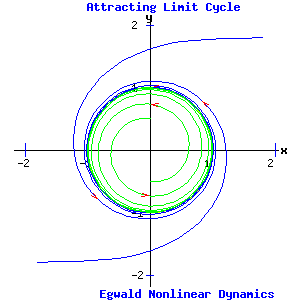μ = - .2   <   0 μ = .2   >   0

Polar Coordinates

Transforming the nonlinear system of differential equations:

dx / dt = f(x, y),         (4)

dy / dt = g(x, y),         (5)

to polar coordinates can reveal the presence of limit cycles.

Let:

x = r * cos(θ),

y = r * sin(θ)

where r and θ are functions of time, t.

Consider the transformation G: (r, θ) → (x, y) defined by (Markley 70-72):

(x,  y)T = G(r, θ) = ( r * cos(θ),  r * sin (θ) )T,       (6)

where "T" indicates the transpose of the vector from a column vector to a row vector.

The Jacobian matrix of G is:

JG(r, θ) =
 cos( θ ) -r * sin( θ ) sin( θ ) r * cos( θ )

Differentiating (6) with respect to time and using the chain rule yields:

d (x,  y)T / dt = JG(r, θ) * d (r,  θ)T / dt.

Multiplying by the inverse of JG(r, θ), and substituting the relations in equations (4) and (5):

d (r,  θ)T / dt = JG-1(r, θ) * ( f(r * cos(θ),  r * sin(θ)),  g(r * cos(θ),  r * sin(θ)) )T

The trajectory ( r(t),  θ(t) ) is a solution of the transformed system, if and only if the trajectory ( r(t) * cos(θ(t)),  r(t) * sin(θ(t)) ) is a solution of the original system.

Example:

In the example above, the transformation to polar coordinates produces:

 dr /dt dθ / dt
=
 cos(θ) sin(θ) -1/r * sin(θ) 1/r * cos(θ)
*
 -r * sin(θ) + μ * r * (1 - r2) * cos(θ) r * cos(θ) + μ * r * (1 - r2) * sin(θ)

Working through this matrix * vector operation and simplifying, the transformed system in polar coordinates is:

dr / dt = μ * r * (1 - r2),         (7)

dθ / dt = 1.                         (8)

The polar transformation has produced a pair of decoupled differential equations.

Equation (7) has fixed points at r1* = 0 , corresponding to (x*, y*) = (0, 0), and r2* = 1, corresponding to the limit cycle.

For 0 < r < 1, dr / dt > 0 if μ > 0, and dr / dt < 0 if μ < 0. For r > 1, dr / dt < 0 if μ > 0, and dr / dt > 0 if μ < 0.

The van der Pol Oscillator

The van der Pol oscillator's dynamics are governed by the 2nd order differential equation:

d2x / dt2 + μ * (x2 - 1) * dx / dt + x = 0,         (9)

where x is the position coordinate, and μ is a positive parameter.

Equation (9) can be written as a system of two first order differential equations:

dx / dt = y,                                 (10)

dy / dt = μ * (1 - x2) * y - x.           (11)

where y is measured as the change in the position coordinate per second.

This system has a fixed point at (x*, y*) = (0, 0).

The Jacobian matrix:

J(x, y) =
 0 1 -2 * μ * x * y - 1 μ * (1 - x2)

evaluated at the fixed point:

J(0, 0) =
 0 1 - 1 μ

has p = trace(J(0, 0)) = μ, and q = det(J(0,0)) = 1 > 0. With μ > 0, the origin is a repeller. If this system has a limit cycle surrounding the origin, it will be an attracting limit cycle, as the following diagrams confirm.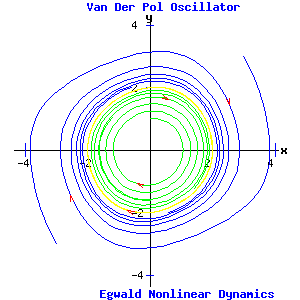μ = .1 μ = .3

For the van der Pol equation, the shape and size of the limit cycle depend on the parameter μ.

Limit Cycles Absent

Various criteria establish that a system does not have a limit cycle.

Describe the differential equation system:

dx1/dt = f1(x1, x2),

dx2/dt = f2(x1, x2),

in vector notation as:

d x / dt = f( x ),

x = (x1, x2).

Gradient Systems

If a continuously differentiable, single-valued, scalar function V(x) exists, whereby the system can be expressed in terms of its gradient:

gradient V = ΛV = (Vx1,  Vx2), as

dx / dt = - ΛV(x),

the system has no closed orbits (and no limit cycle).

Liapunov Functions (Lorenz 36)

Let x* be a fixed point of the system. If a real-valued (Liapunov) function V(x) exists on the domain of the system, such that:

 V(x*) = 0 and V(x) > 0  if x ≠ x*, and dV(x) / dt ≤ 0,  for x ≠ x*

then x* is stable. Moreover, if:

 dV(x) / dt < 0,  for x ≠ x*

then x* is a globally, asymptotically stable fixed point. Thus, the system has no closed orbits (and no limit cycle).

Dulac's Criterion (Strogatz 202)

Suppose the domain of the differential system is a simply connected subset D of the plane R2. If a continuously differentiable, real-valued function g(x) defined on D exists, such that the gradient of the product of g(x) with f(x) ,   Λ * (g(x)*f(x)),  has one sign throughout D, then the system has no closed orbits (and no limit cycle) enclosed in D.

Limit Cycles Present

A region of the plane is called annular if it is topologically similar to a flat donut. If the direction field of a system is oriented inwards along the boundary of an annular region, and if the region contains no fixed points, a limit cycle is present in the annular region. In the next diagram, the two concentric olive circles form the boundaries of the annular region. All direction field arrows crossing these boundaries point into the annular region.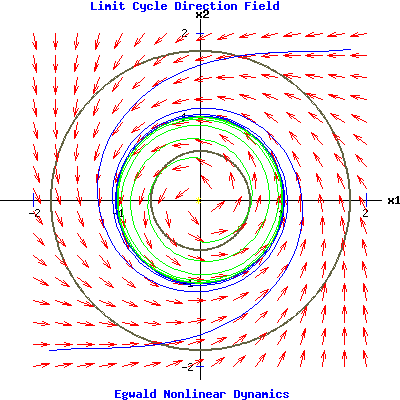If the direction field of the system is oriented inwards along the boundary of an annular region, the region is called a trapping region. If the trapping region excludes fixed points, it contains a limit cycle.

The Poincaré-Bendixson Theorem

Let the component functions of the differential system, f = (f1, f2)T, have continuous first partial derivatives. If the set R, the union of an annular region and its boundary, contains no fixed points, but contains a solution trajectory of the system for all time t > T, then R contains a limit cycle.

Kaldor Business Cycle Model (Lorenz 43-47)

Nicholas Kaldor investigated the interaction between investment and savings to produce cyclical movements in income and capital. In his model, investment and savings are functions of the flow of income, y, and the stock of capital, k, with capital depreciating at a constant rate, δ. The flow of income equals the value of the production of consumer and capital goods. The dynamics of the system are governed by:

dy /dt = f(y, k) = α * ( I(y, k)  -  S(y, k) ),         (12)

dk / dt = g(y, k) = I(y, k)  -  δ * k,                  (13)

Equation (12) describes the dynamics of income, which increases when investment, I(y, k), exceeds savings, S(y, k), and vice versa. Equation (13) describes the growth of the stock of capital, equalling the production of capital goods, I(y, k), less depreciation, δ * k. The parameter α in equation (12) determines the speed at which the aggregated market for capital and consumer goods adjusts.

Suppose (ye, ke) is the fixed point of the Kaldor system, so that:

f(ye, ke) = α * ( I(ye, ke)  -  S(ye, ke) ) = 0,

g(ye, ke) = I(ye, ke)  -  δ * ke = 0.

The next diagram graphs investment, savings, and their difference , dy, as functions of income, y, for capital at k = ke, with α = 4 .  As shown in the diagram, investment and savings are increasing functions of income, y. Furthermore, the slope of the investment function, Iy, increases for y < ye, while Iy decreases for y > ye. The amplitude of dy depends on α.

 Income-Goods Market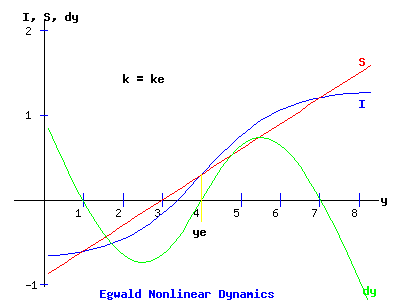For low values of y, savings are negative as people liquidate wealth. Investment is also negative for low values of y. Capital (machinery and equipment) taken out of the production processes may be too obsolete, costly, or unprofitable to put back on-line when demand for product increases. Furthermore, new product lines may require new machinery and equipment as demand for consumer goods increases as income increases. (Kaldor assumed that investment is positive for all y > 0.)

In the spirit of Kaldor, the functions I(y, k) and S(y, k) are restricted as follows:

 Iy > 0;  Sy > 0;  Ik < 0;  Sk > 0 Iyy > 0 for y < ye, and Iyy < 0 for y > ye Iy(ye, ke) > Sy(ye, ke)

With these assumptions, the phase portrait and the y and k nullclines appear as follows: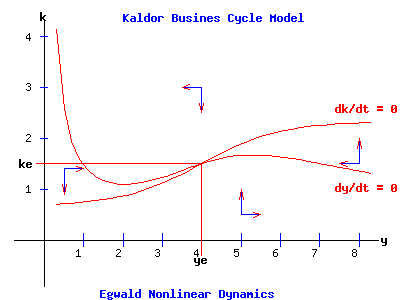The stability of the fixed point depends on the Jacobian of the system:

J(y, k) =
 α * (Iy - Sy) α * (Ik - Sk) Iy Ik - δ

evaluated at the fixed point (ye, ke).

For the specific functions used here , (ye, ke) = (4, 1.5), and the Jacobian is:

J(y, k) =
 α * (1/2 - 3/10) α * (-1 - 1/5) 1/2 -1 - 1/5

Consequently, the systems stability is determined by:

 p = trace(J) = α * (Iy - Sy) + (Ik - δ) = 1/5 * α - 6/5, q = det(J) = α * (Iy - Sy) * (Ik - δ) - Iy * α * (Ik - Sk) = 4/25 * α > 0

The sign of p is negative for α < 6, and positive for α > 6.

For α < 6, the fixed point is an attractor, as shown in the next diagram:

 α = 4For α > 6, the fixed point is a repeller. Moreover, as shown in the next diagram a limit cycle emerges:

 α = 8Kaldor Model Hopf Bifurcation

The fixed point of the Kaldor model loses its stability as α, the adjustment coefficient of the income-goods market equation, increases past α = αc = 6. The fixed point switches from attractor to repeller, and a limit cycle emerges for α > 6.

 α = 6Value of parameter α: 6

Use the form to set the value of α for the Kaldor business cycle model, 1 ≤ α ≤ 12.

References.

• Boyce, William E. and Richard C. DiPrima. Elementary Differential Equations and Boundary Value Problems. New York: John Wiley, 1997.
• Burden, Richard L. and J. Douglas Faires. Numerical Analysis. 6th ed. Pacific Grove: Brooks/Cole, 1997.
• Demmel, James W. Applied Numerical Linear Algebra. Philadelphia: Siam, 1997.
• Devaney, Robert L. An Introduction to Chaotic Dynamical Systems. Menlo Park, CA: Benjamin/Cummings, 1986.
• Elaydi, Saber N. Discrete Chaos. Boca Raton: Chapman & Hall, 2000.
• Erdi, Péter. Complexity Explained. Berlin: Springer, 2007
• Kaplan, Wilfred. Ordinary Differential Equations. Reading: Addison-Wesley, 1958.
• Lorenz, Hans-Walter. Nonlinear Dynamical Economics and Chaotic Motion. Berlin: Springer-Verlag, 1993.
• Markley, Nelson G. Principles of Differential Equations. Hoboken, N.J.: John Wiley, 2004.
• Mathews, John H. and Kurtis D. Fink. Numerical Methods Using MATLAB. 3rd ed. Upper Saddle River: Prentice Hall, 1999.
• Nagata, K. Wayne. Nonlinear Dynamics and Chaos: Mathematics 345 Lecture Notes. Vancouver: University of B.C., 2006.
• Puu, Tönu. Attractors, Bufurcations, and Chaos: Nonlinear Phenomena in Economics. Berlin: Springer, 2000.
• Strang, Gilbert. Linear Algebra and Its Applications. 3d ed. San Diego: Harcourt, 1976.
• Strogatz, Steven H. Nonlinear Dynamics and Chaos. Cambridge MA: Perseus, 1994.
• Watkins, David S. Fundamentals of Matrix Computations. New York: John Wiley, 1991.Copyright © Elmer G. Wiens:   EgwaldWeb ServicesAll Rights Reserved.    Inquiries